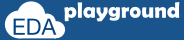# How to randomize a variable in SystemVerilog?

SystemVerilog provides multiple methods to generate random data. By using any of these methods a variable can be randomized.

## Systemverilog randomization methods

• \$urandom( ) and \$random( )
• \$urandom_range( )
• std::randomize():
• randomize():

### \$urandom( ) and \$random( )

The \$urandom( ) function returns a new 32-bit random number

`variable = \$urandom(seed); //seed is an optional argument`

\$random() is same as \$urandom() but it generates signed numbers

### \$urandom_range( )

The \$urandom_range() function returns an unsigned integer within a specified range.

`variable = \$urandom_range( int unsigned maxval, int unsigned minval = 0 );`

Below example shows the usage of urandom and urandom_range.

```module system_funcations;
bit [31:0] data;
```

```  initial begin
data  = \$urandom * 6;

addr2 = \$urandom_range(20); //takes max value as '0'
addr3 = \$urandom_range(20,30); //considers max value as '30' and min value as '20'
```
```    \$display("addr1=%0d, addr2=%0d, addr3=%0d",addr1,addr2,addr3);
end
```
```endmodule
```

Simulator Output:

Execute the above code on### std::randomize():

Variables can be randomized by using std::randomize method. It can accept the inline constraints using the “with” clause.

`std::randomize (variable);`

Can also be used as below,

```std::randomize (variable);
std::randomize (variable) with { constraint's; };

std::randomize (variable-1, variable-2 ... variable-n);
std::randomize (variable-1, variable-2 ... variable-n) with { constraint's; };```

Below example shows the usage of std::randomize().

```program std_randomize;
bit [31:0] data;
bit [63:0] data_x_4;

initial begin

std::randomize(data,data_x_4) with { data     == addr * 8;
data_x_4 == data * 4;
};

\$display("Value of data is %0d",data);
\$display("Value of data_x_4 is %0d",data_x_4);
end
```
```endprogram
```

Simulator Output:

Execute the above code on